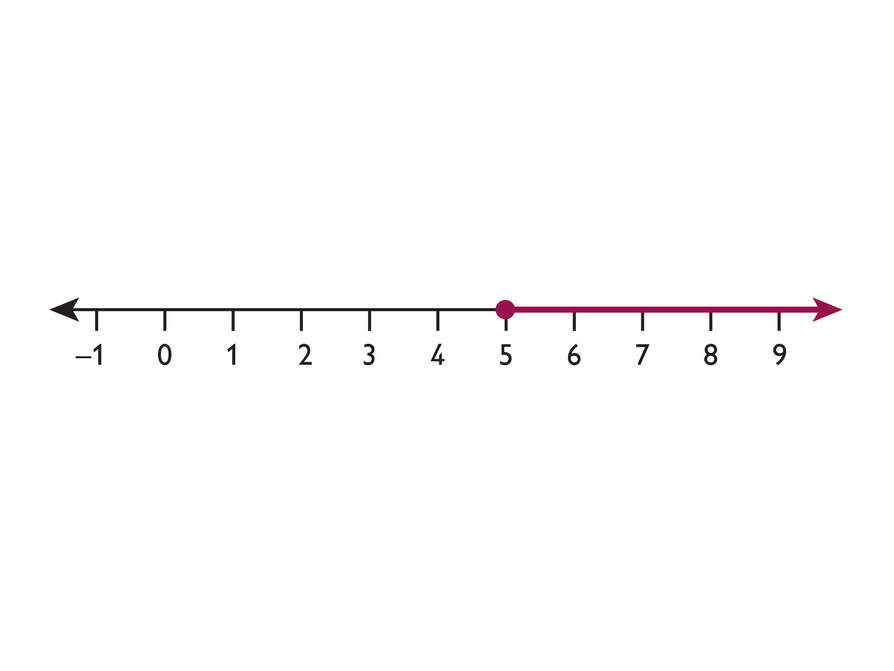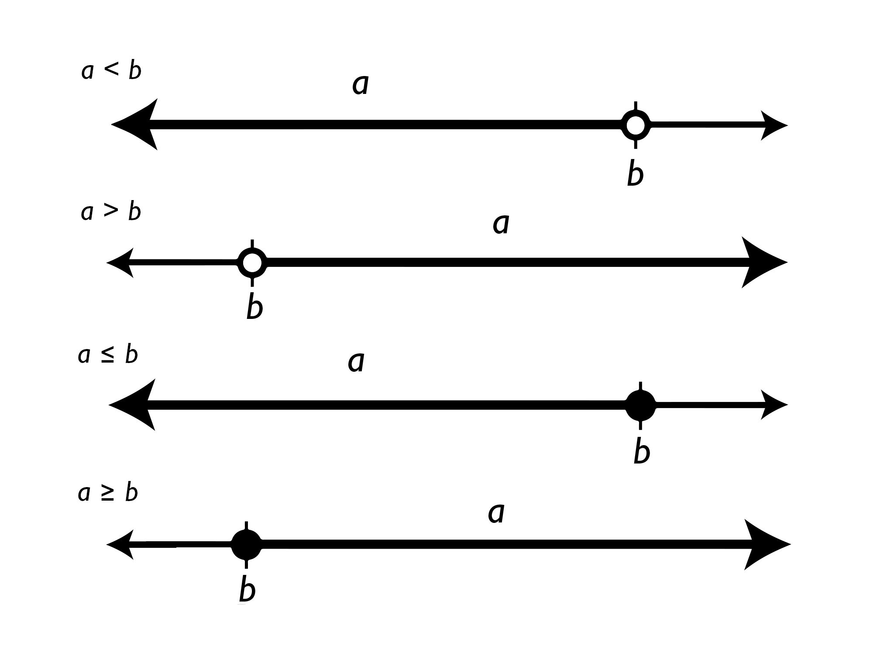# Representing An Inequality On A Number Line# Match Inequalities to Graphs

• Match each inequality to a number line.
• Write the inequality in words in the space provided; for example, "x is less than 5."

INTERACTIVE: Match Inequalities to Graphs

## Opening

Represent inequalities on a number line and find at least one value that makes the inequality true.

# Inequalities and Number Lines

For each inequality do the following.

• Represent the inequality on a number line using the number line interactive.
• Write one value that makes the inequality true.
• Write the inequality using words.
1. x ≥ –2
2. x < 4
3. 12 ≥ x
4. x ≤ −3.5
5. x < 1.5

INTERACTIVE: Inequalities and Number Lines

## Hint:

• Where is the number located on the number line?
• Should the starting point be a closed circle or an open circle?
• What is one value that makes the inequality true? Is that value shaded on the number line?

# Write About the Number Line

• Write about a real-world situation that could be represented by this number line.## Hint:

Think about situations involving age, money, or weight.

# Prepare a Presentation

• Explain how you represented an inequality on a number line.

# Challenge Problem

Denzel says that because 2<5, that means −2<−5.

• Do you agree? Explain why or why not.

# Ways of Thinking: Make Connections

Take notes about your classmates’ representations of inequalities on a number line.

## Hint:

• Why is the circle filled in?
• Why is the circle open?
• Why does the line go in that direction?
• Explain why –4 is less than –3.5.
• What is the greatest number that x could be?

# Summary of the Math: Inequalities

• An inequality is a relationship that holds between two values that are different.

• a < b means that a is less than b (or, equivalently, that b is greater than a).

• a > b means that a is greater than b (or, equivalently, that b is less than a).

• ab means that a is less than or equal to b (or, equivalently, that b is greater than or equal to a).

• ab means that a is greater than or equal to b (or, equivalently, that b is less than or equal to a).## Hint:

Can you:

• Explain the meaning of each inequality symbol?
• Show how each type of inequality is represented on a number line?

# Solve and Use Equations

Complete this Self Check by yourself.

For each equation do the following.

• Solve the equation. Name the property of equality you use and show each step.
• Write and solve a word problem that each equation could represent.
1. x + 5 = 17
2. 4x = 22
3. $\frac{x}{6}=\frac{5}{8}$